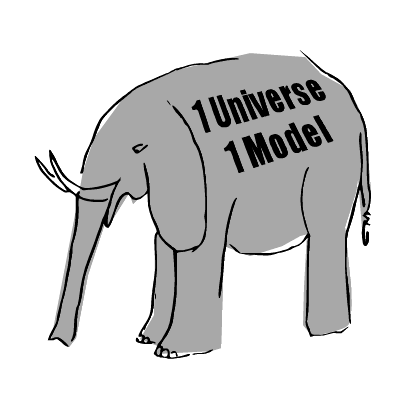# Four Forces – A Dissident Perspective

It is probably the most sought after prize in physics. Einstein wanted it, everyone talks about it, and dissidents claim to have solved it. What is it? The unification of the four forces in nature. They are:

1. Gravity
2. Electromagnetism
3. Strong Force (what keeps atomic nucleus together)

The latest in this quest for the “grand unification” has been quantum gravity or the unified field theory where physicists are trying to unify Einstein’s general relativity with the strong force inside the atom. So far, there has been little success with many candidate theories not passing the grade and having been debunked by the experiments during the last 10 years of the Large Hadron Collider.

I’m here to tell you today, that the four forces of nature exist but the goal of unification is an artificial problem that has resulted in the way we have advanced science during the last 400 years.

### Isolated Areas of Study

Human interest in gravity, electromagnetism, chemistry, and eventually particle physics have created their own worlds, own vocabulary, and own empirical equations. Concepts from each of the areas seemed different enough that they were thought to be elementary in nature.

Gravity has the graviton. Electromagnetism has charge, chemistry has beta decay, and particle physics has a virtual zoo of supposedly fundamental properties that have no relationship with the other areas.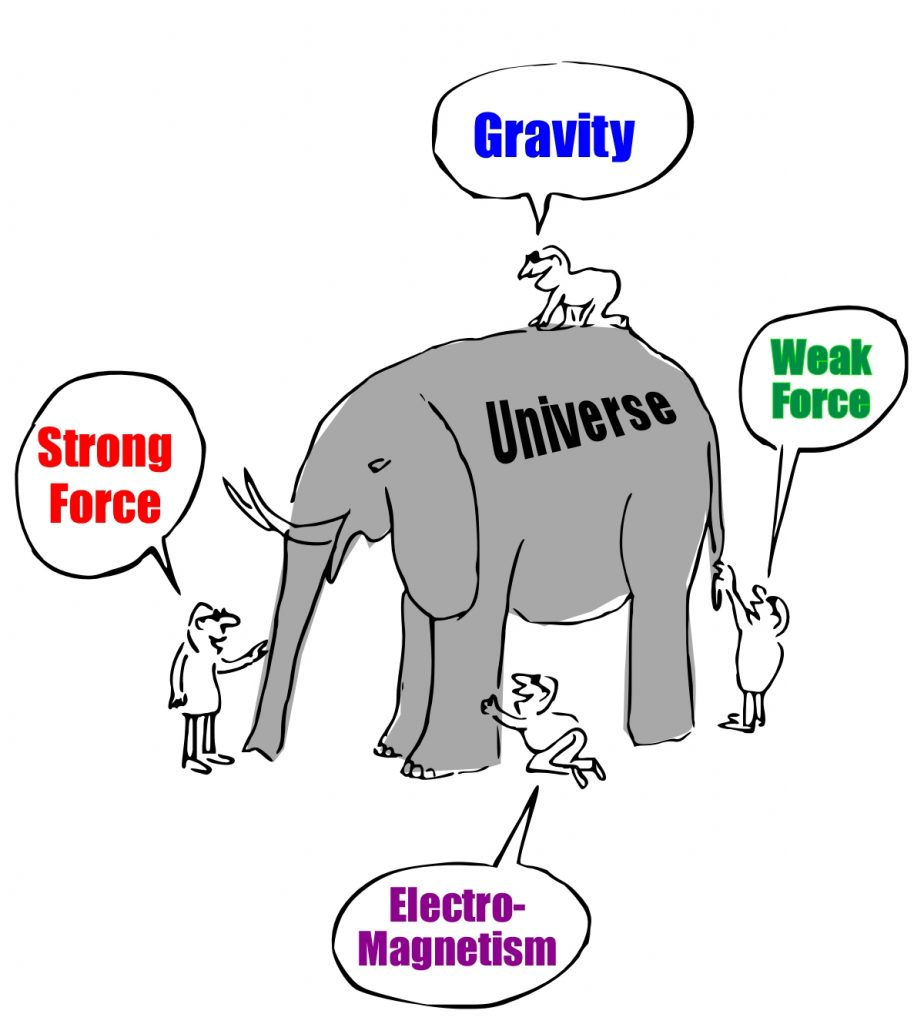Areas of study in physics have been isolated from each other creating the artificial need for unification

In a world of blind physicists touching different parts of the universe, the urge to find a description of the entire elephant is a natural human tenancy. And there are many attempting to describe the entire elephant.

### Force Equations

Science has relied heavily on mathematics. The equations produced by physicists and scientists in each of these separate areas are equations that try to describe how the universe functions. Inevitably, the equations end up as force equations where movements can be described and predicted.

So it is logical that the force equations are the first place where scientists look in order to find a theory of “everything” or as they often say: unify the forces.

### Failure of Big Physics

Mainstream physics has not been able to accomplish this unification. Physicists currently have tasked themselves with unifying Einstein’s general relativity and the strong force. Quantum gravity or gravity at the subatomic level is calling physicists like sirens of old calling sailors to their deaths along the rocky coast. But in this case, the coast is particle physics and relativity.

But as the science woke community knows, this endeavor is doomed given the inherent and fundamental problems with non-physical models such as general relativity and the Standard Model. Unifying two fundamentally flawed concepts is a wasted endeavor.

Luckily for you and for the human race, we have amazing people out there tackling this problem from all angles, each one worth our time to examine.

## Three Example Models

New models and theories of the universe can be broken down into three groups: equation unification, equation and new mechanisms, and ones that simply propose new mechanisms without equations.

### Unifying the Force Equations

When it comes to unifying the actual force equations of mainstream science, we need look no further than science woke and the award-winning Dr. Bill Lucas, a physicist working outside the mainstream who has come up with a universal force equation.

In his book, “The Universal Force”, Dr. Lucas describes an equation that in fact unifies all four forces of the universe through axiomatic and empirical scientific methods.

Dr. Lucas writes on the back of his book “The Universe Force Volume 1”:

The resulting classical electrodynamic force law obtained appears capable of explaining all the phenomena for which relativity theory and quantum mechanics were invented to explain”.

Dr. William Lucas, The Universal Force Volume 1

As you can see here, Dr. Lucas’ equation is quite complex but in fact, does what mainstream science could not do: come up with one force equation for the universe. Unfortunately, Dr. Lucas is a vocal critic of Einstein’s theory of relativity and therefore is shunned by mainstream physics without anyone in mainstream physics looking at his work.

### Unifying Equations and New Model

A recent new comer to the science woke community, Jeff Yee, has been working on his Energy Wave Model which is an example where he both unifies existing energy and force equations while proposing a new physical model. In Yee’s model, there are only two particles: aether granules and neutrinos.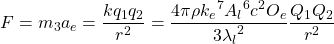Yee then goes on to describe particles in the standard model as standing waves in aether centered around a neutrino core. He proposes a new periodic table of the current subatomic particles in the standard model and is able to derive these particles and their properties with only 4 constants instead of the 19 or more constants of mainstream science.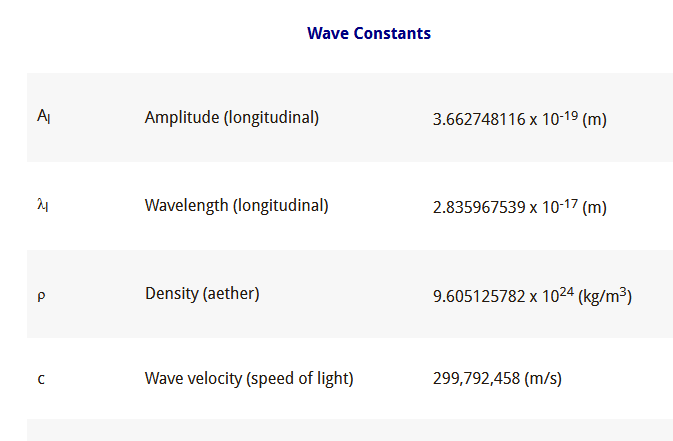The Energy Wave Theory only has four constants as compared with 19 or more in the Standard Model.

Jeff’s work is extensive and exhaustive and he has been producing videos on his YouTube channel together with a fantastic website where he guides people through his model which is a great step forward in simplifying the existing standard model.

His model is well worth you time to investigate and represents both a simplification in math as well as in the physical model for the universe.

There are two models I want to talk about that are proposing new mechanisms for the universe which do not attempt to unify the supposed four forces of nature. They attempt to describe the universe with new mechanics without working with empirical equations. They rely on Newtonian mechanics and infinity.

### Infinite Universe Theory

The infinite universe theory is a theory by scientist and philosopher Dr. Glenn Borchardt who proposes that aether is the dominant feature in a universe of infinite levels. Aether in his model relies on strictly Newtonian laws with light being waves in aether and gravity from an entrained aether around bodies. The other two forces as well are assumed to not be unique but it is expected that his model when developed further will indeed describe the four forces.

Although Dr. Borchardt has not gone into the description of the actual construct of atoms as Yee and Lucas have, Borchardt has replaced the electron, light, and gravity with an aether particle. This is a great simplification in the mechanics of the universe reducing it to moving particles and Newtonian forces. He calls the mechanics of his model: neomechanics.

In his most recent book the “Infinite Universe Theory”, Borchardt reveals one of his hallmark concepts in the new age of the scientific woke: the concept of infinity. His aether is not an ultimate particle in the universe. There are aethers in his model all the way down with Borchardt labeling them A1, A2, A3, and on down. He also says that there are macro structures infinitely above. This avoids the problem of ever having magical particles with magical properties.

In the end, Borchardt’s model does not look at all into the empirical equations of the supposed “four forces”, but instead, proposes an entirely new physical model.

### The Particle Model

The particle model, like the Borchart model is completely based on Newtonian physics and also have infinite levels. The model describes all four forces are being a result of simple Newtonian mechanics. The difference is that light in the particle model is not through collision as it is with aether, but are formed as waves of same-speed particles traveling in waves.

What is interesting in this model is how Coulomb’s force equation can simply be replaced by the same equation for Newton’s gravity. The only difference is, it involves gravity particles at the next level. Just as in Borchardt’s aether model where there are aethers at all levels of the universe, in the particle model, there are gravity particles at all levels of the universe.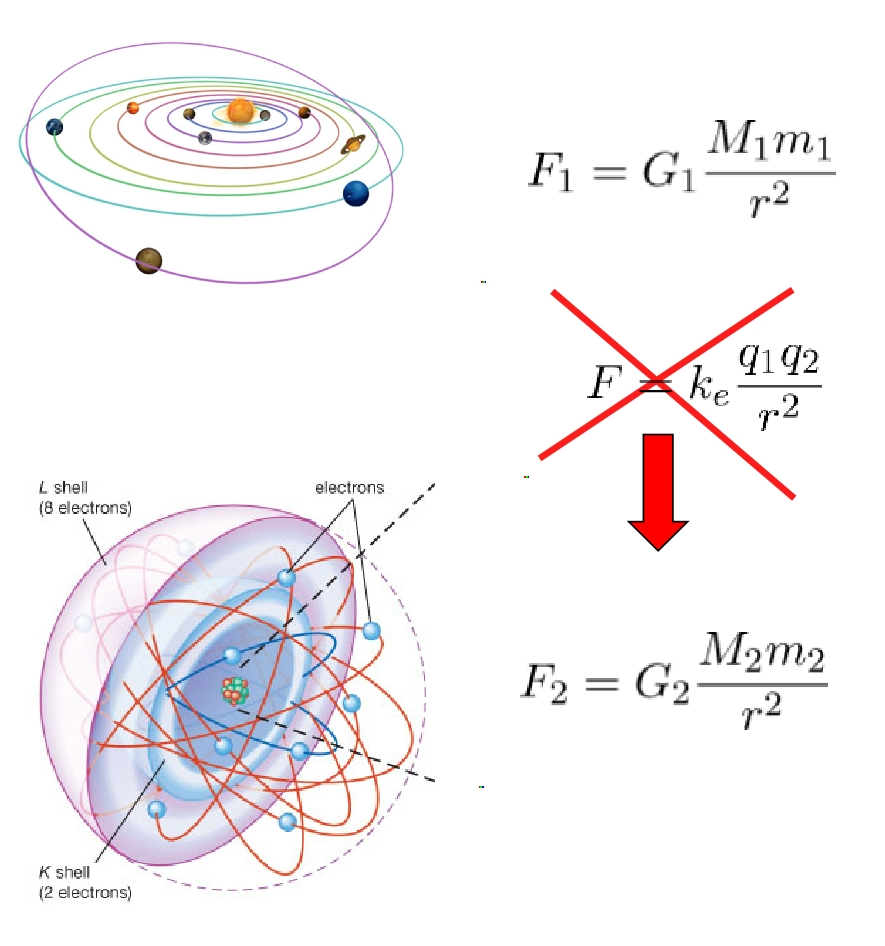The Particle Model uses gravity at two levels using the same principle replacing at least two forces with one generic field of particles

Notice how the empirical equations for Coulomb’s law is in fact in the same form as Newton’s equation for gravity. In the particle model, gravity as we know it is caused by G1 particles. Whereas what is called Coulomb’s force is in the particle model, gravity caused by G2 particles at a different level.

Gravity is the result of a particular behavior of similar particles no matter what level of the universe we are examining. In the Particle Model, gravity is caused by similar particles traveling in random directions.

The G1 particle replaces the photon, electron, electricity, and magnetic fields, thus greatly simplifying the standard model. Like the Borchardt model, it has infinite levels and only works with Newtonian concepts.

## Equations Come from the Models

Modern models differ from the Standard model in their approach to what is called “unifying the four forces”. Whereas the Standard model tries to glue together the four separately discovered equations as separate and distinct forces, newer models from the dissident community are creating models that describe the universe not through forces or equations, but through physicality and behaviors.

Whether it be a truly unified force equation like Dr. Lucas’ Universal Force Law, a hybrid model like Jeff Yee’s Energy Wave theory with equation simplification and a new model, or completely equation-free models like the Infinite Universe Model or the Particle Model, new scientists working outside the mainstream are making progress where mainstream is not.

And you can read about this progress on our website ScienceWoke.org where you can find critical thinkers taking science forward into the 21st century.